# 52 Enter Calc Functions

 <  Day Day Up  >

Computing totals is so common, Calc makes the Sum() function even easier to use by placing the Sum button on the Function toolbar. Just click a blank cell below or to the right of a range of values, click Sum , and Calc computes the sum and writes the proper Sum() function for you.

Before You Begin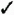51 About Calc Functions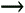53 Use the Function AutoPilot

You'll have to type the remaining functions yourself when you want to use them, but doing so is far from a chore. 51 About Calc Functions lists some of the most common functions you'll use. For the rest, use Calc's Help feature to find the function you want to use and then type that function and its arguments to get the result you want.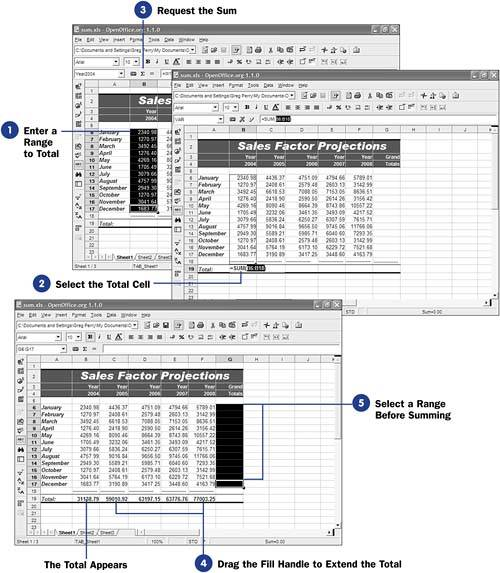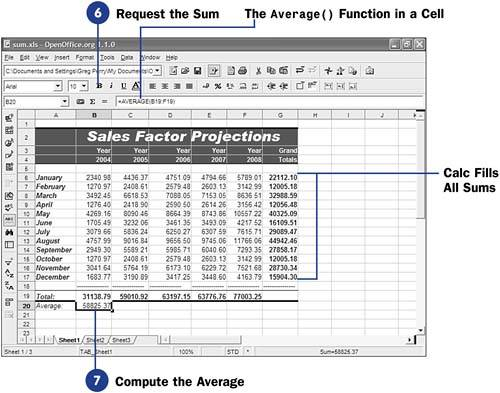1. Enter a Range to Total

Type the range of values you want to get a total of. The range can be a row or a column of numbers .

2. Select the Total Cell

Click to select the cell that you want to hold the total.

3. Request the Sum

Click the Function toolbar's Sum button. Calc analyzes the current cell and guesses at the row or column that is to comprise the sum. For example, Calc might highlight the column of cells above the current cell and indicate its range in the Sum() function.

If the range is correct, press Enter to accept the sum; otherwise , type a different range inside the Sum() function's argument list and then press Enter . The sum then appears in the cell.

NOTE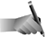As with formulas, you can select a cell containing a function and press F2 to change the function, its arguments, or a formula that uses the function.
4. Drag the Fill Handle to Extend the Total

As with any other formula or cell label, you can drag the formula's fill handle, whose cell contains a function such as Sum() , to another cell to extend that formula.

5. Select a Range Before Summing

Calc doesn't make you request one sum at a time. Just select all the cells at the end of a set of rows or columns before clicking Sum .

6. Request the Sum

When you click the Sum button, Calc automatically sums the entire column or row you selected.

7. Compute the Average

Although other functions aren't represented on the Function toolbar, you can easily type functions that you need. To get a yearly sales average, for example, you can type =Average(B19:F19) in cell B20 to learn your average projected sales over the next 5 years .

 <  Day Day Up  >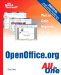Sams Teach Yourself OpenOffice.org All In One
ISBN: 0672326183
EAN: 2147483647
Year: 2003
Pages: 205
Authors: Greg Perry

Similar book on Amazon